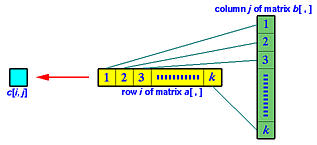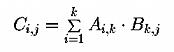# Problem: Matrix MultiplicationWe discussed one way of multiplying two matrices using threads on a previous page. Given two matrices Am×k (m rows and k columns) and Bk×n (k rows and n columns), we want to compute the product of A and B into a matrix C of m rows and n columns. The entry of C on row i and column j is the sum of the products of the corresponding elements on row i of matrix A and column j of matrix B as shown below:More precisely, Ci,j is computed with the following formula:### Problem

Write a program that reads in a m×k array and a k×n array. Then, create m×n threads, one for each entry Ci,j to compute its value. Finally, prints the result. Note that you should aim for maximum parallelism. More precisely, all created threads should run concurrently.